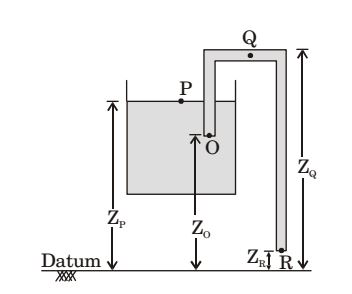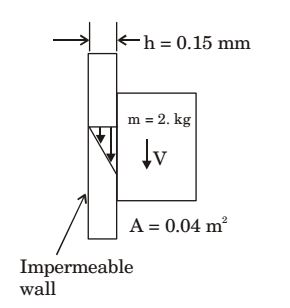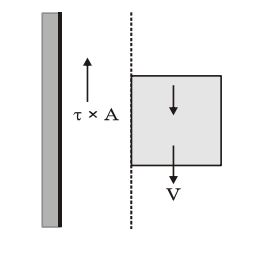## Fluid Mechanics and Hydraulic Machinery Miscellaneous

#### Fluid Mechanics and Hydraulic Machinery

1. A centrifugal pump is required to pump water to an open water tank situated 4 km away from the location of the pump through a pipe of diameter 0.2 m having Darcy's friction factor of 0.01. The average speed of water in the pipe is 2 m/s. If it is to maintain a constant head of 5 m in the tank, neglecting other minor losses, the absolute discharge pressure at the pump exit is

1. Given: d = 0.2 m, L = 4000 m
f = 0.01, v = 2 m/s

 hf = fLv² = 0.01 × 4000 × (2)² 2gd 2 × 9.81 × 0.2

= 40.77 m
= ρg(hf + h + hatm)
= 1000 × 9.81(40.77 + 5 + 10.3)
= 5.50 × 105 N/m² = 5.50 bar

##### Correct Option: B

Given: d = 0.2 m, L = 4000 m
f = 0.01, v = 2 m/s

 hf = fLv² = 0.01 × 4000 × (2)² 2gd 2 × 9.81 × 0.2

= 40.77 m
= ρg(hf + h + hatm)
= 1000 × 9.81(40.77 + 5 + 10.3)
= 5.50 × 105 N/m² = 5.50 bar

1. Water at 25°C is flowing throug a 1.0 km long G.I. pipe of 200 mm diameter at the rate of 0.07 m³/s. If value of Darcy friction factor for this pipe is 0.02 and density of water is 1000 kg/m³, the pumping power (in kW) required to maintain the flow is

1.  v = 0.07 = 2.23 m/s π/4(0.2)²

 Head loss, hf = 4fLv² = 2.23 m/s d × 2g

 = 4 × 0.02 × 1000 × (2.23)² = 25.35 0.2 × 2 × 9.81

∴Power = ρghf
= 9.81 × 0.07 × 25.35 = 17.4 kW

##### Correct Option: B

 v = 0.07 = 2.23 m/s π/4(0.2)²

 Head loss, hf = 4fLv² = 2.23 m/s d × 2g

 = 4 × 0.02 × 1000 × (2.23)² = 25.35 0.2 × 2 × 9.81

∴Power = ρghf
= 9.81 × 0.07 × 25.35 = 17.4 kW

1. A siphon is used to drain water from a large tank as shown in figure below. Assume that the level of water is maintained constant. Ignore frictional effect due to viscosity and losses at entry and exit. At the exit of the siphon, the velocity of water is1. Applying Bernoulli’s equation between the points ‘P’ and ‘R’.

 Patm + V²P + Zp = Patm + V²P + ZR 2g ρg 2g ρg

Since the level of liquid in tank remains the same,
VP = 0
∴ VR = √2g(ZP - ZR)

##### Correct Option: B

Applying Bernoulli’s equation between the points ‘P’ and ‘R’.

 Patm + V²P + Zp = Patm + V²P + ZR 2g ρg 2g ρg

Since the level of liquid in tank remains the same,
VP = 0
∴ VR = √2g(ZP - ZR)

1. The viscous laminar flow of air over a flat plate results in the formation of a boundary layer. The boundary layer thickness at the end of the plate of length L is δL. When the plate length is increased to twice of its original length, the percentage change in Iaminar boundary layer thickness at the end of the plate (with respect to δL) is ________

1. As per Blausius equation,

 ρ = 5x = 5x √Rex √ρV∞.x/μ

ρ = ∝ √x
ρL ∝ √L
ρ2L ∝ √2L
 ρ2L - ρL 100 =√2L - √L× 100 ρL √L

= (√2 - 1) × 100 = 41.42%

##### Correct Option: B

As per Blausius equation,

 ρ = 5x = 5x √Rex √ρV∞.x/μ

ρ = ∝ √x
ρL ∝ √L
ρ2L ∝ √2L
 ρ2L - ρL 100 =√2L - √L× 100 ρL √L

= (√2 - 1) × 100 = 41.42%

1. A solid block of 2.0 kg mass slides steadily at a velocity Valong a vertical wall as shown in the figure below. A thin oil film of thickness h = 0.15 mm provides lubrication between the block and the wall. The surface area of the face of the block in contact with the oil film is 0.04 m². The velocity distribution within the oil film gap is linear as shown in the figure. Take dynamic viscosity of oil as 7 × 10-3 Pa-s and acceleration due to gravity as 10 m/s². Neglect weight of the oil. The terminal velocity V (in m/s) of the block is __________(correct to one decimal place).1.Terminal velocity is a constant velocity i.e. the net acceleration is zero.
So, ΣFnet = ma
mg – τA = 0
τA = mg

 μ V A = mg h

 7 × 10-3 × V × 0.04 = 2 × 10 0.15 × 10-3

V = 10.714 m/s

##### Correct Option: ATerminal velocity is a constant velocity i.e. the net acceleration is zero.
So, ΣFnet = ma
mg – τA = 0
τA = mg

 μ V A = mg h

 7 × 10-3 × V × 0.04 = 2 × 10 0.15 × 10-3

V = 10.714 m/s